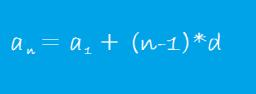# Arithmetic 2390

Determine the first 5 members of the arithmetic sequence if a6 = -42, d = -7

a1 =  -7
a2 =  -14
a3 =  -21
a4 =  -28
a5 =  -35

### Step-by-step explanation:Did you find an error or inaccuracy? Feel free to write us. Thank you!